## Example Questions

### Example Question #1 : How To Find A Line On A Coordinate Plane

Billy set up a ramp for his toy cars. He did this by taking a wooden plank and putting one end on top of a brick that was 3 inches high. He then put the other end on top of a box that was 9 inches high. The bricks were 18 inches apart. What is the slope of the plank?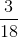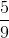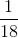Explanation:

The value of the slope (m) is rise over run, and can be calculated with the formula below: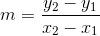The coordinates of the first end of the plank would be (0,3), given that this is the starting point of the plank (so x would be 0), and y would be 3 since the brick is 3 inches tall.

The coordinates of the second end of the plank would be (18,9) since the plank is 18 inches long (so x would be 18) and y would be 9 since the box was 9 inches tall at the other end.

From this information we know that we can assign the following coordinates for the equation: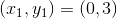and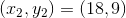Below is the solution we would get from plugging this information into the equation for slope: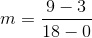This reduces to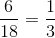### Example Question #12 : New Sat Math Calculator

What is the slope of the line depicted by the graph?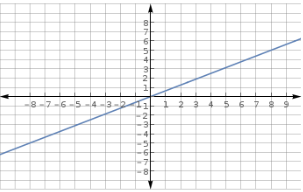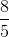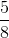Explanation:

Looking at the graph, it is seen that the line passes through the points (-8,-5) and (8,5).The slope of a line through the points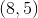and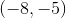can be found by setting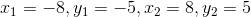:

in the slope formula: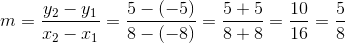### Example Question #21 : Graphing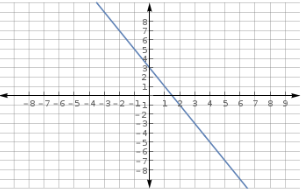What is the-intercept of the function that is depicted in the graph above?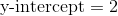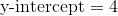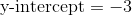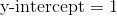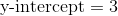Explanation:

This question tests one's ability to recognize algebraic characteristics of a graph. This particular question examines a linear function.

Knowing the standard and the concept for which it relates to, we can now do the step-by-step process to solve the problem in question.

Step 1: Identify the general algebraic function for the given graph.

Since the graph is that of a straight line, the general algebraic form of the function is,where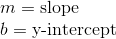Step 2: Identify where the graph crosses the-axis.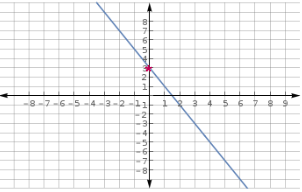Therefore the general form of the function looks like,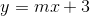The-intercept is three.

### Example Question #52 : New Sat Math Calculator

The equation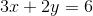represents a line.  This line does NOT pass through which of the four quadrants?

III

Cannot be determined

IV

II

I

III

Explanation:

Plug inforto find a point on the line: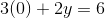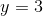Thus,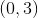is a point on the line.

Plug inforto find a second point on the line: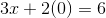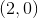is another point on the line.

Now we know that the line passes through the pointsand.

A quick sketch of the two points reveals that the line passes through all but the third quadrant.

### Example Question #671 : Sat Mathematics

A farmer is designing rectangular pen for his cows. One side of the pen will be blocked by a steep hill, and the other three sides of the pen will be fenced off with wire. If the farmer has 20 meters of wire, what is the maximum area of the pen that he can build in square meters?

25
25/2
75/2
75
50
Explanation:

Let l = length and w = width of the pen. Let us assume that the side blocked by the mountain is along the length of the pen.

The length of wire used to make the pen must equal l + 2w, because this is the perimeter of a rectangle, excluding one of the lengths. The area of the pen will equal l x w.

l + 2w = 20

l = 20-2w

A = l x w = (20-2w)(w) = 20w - 4w2

Let A be a function of w, such that A(w) = 20w - 4w2. We want to find the maximum value of A. We recognize that the graph of A must be in the shape of a parabola, pointing downward. The maximum value of the parabola will thus occur at the vertex.

We want to rewrite A(w) in the standard form of a parabola, given by f(x) = a(x-h)2+k. In order to do this, we must complete the square.

20w-4w= -4w2+20w = -4(w2-5w) = -4(w2-5w + 25/4) + 25 = -4(w-5/2)2+25

Thus, the vertex of the parabola occurs at (5/2, 25), which means that w = 5/2.

Going back to our original equation, l + 2(5/2) = 20, and l = 15.

A = l x w = 15(5/2) = 75/2

### Example Question #672 : Sat Mathematics

Which of the following is true about the quadratic function f(x)=(x+4)2 - 3?

The graph is a parabola with two x-intercepts.

The function is one-to-one.

The graph is a parabola whose vertex is (4,-3).

The function is even.

The graph is a parabola with two y-intercepts.

The graph is a parabola with two x-intercepts.

Explanation:

The function is given in vertex form, which is (x-h)2+k where the vertex of the parabola is the point (h,k).  In this particular function, h=-4 and k=3, so the vertex is (-4,-3).  No parabola is one-to-one, as they don't pass the horizontal line test.  While parabolas can be even functions, this will only happen when the vertex is on the y-axis (or when h=0) because even functions must be symmetric with respect to the y-axis.  No function can have two y-intercepts, as it would then not pass the vertical line test and not be a function.  This parabola does have two x-intercepts, however.  This can be shown by setting y=0 and solving for x, or by simply realizing that the vertex is below the x-axis and the parabola opens up.

### Example Question #673 : Sat Mathematics

Let f(x) = ax2 + bx + c, where a, b, and c are all nonzero constants. If f(x) has a vertex located below the x-axis and a focus below the vertex, which of the following must be true?

I. a < 0

II. b < 0

III. c < 0

I and III only

I, II, and III

II and III only

I and II only

I only

I and III only

Explanation:

f(x) must be a parabola, since it contains an x2 term. We are told that the vertex is below the x-axis, and that the focus is below the vertex. Because a parabola always opens toward the focus, f(x) must point downward. The general graph of the parabola must have a shape similar to this:Since the parabola points downward, the value of a must be less than zero. Also, since the parabola points downward, it must intersect the y-axis at a point below the origin; therefore, we know that the value of the y-coordinate of the y-intercept is less than zero. To find the y-coordinate of the y-intercept of f(x), we must find the value of f(x) where x = 0. (Any graph intersects the y-axis when x = 0.) When x = 0, f(0) = a(0) + b(0) + c = c. In other words, c represents the value of the y-intercept of f(x), which we have already established must be less than zero. To summarize, a and c must both be less than zero.

The last number we must analyze is b. One way to determine whether b must be negative is to assume that b is NOT negative, and see if f(x) still has a vertex below the x-axis and a focus below the vertex. In other words, let's pretend that b = 1 (we are told b is not zero), and see what happens. Because we know that a and c are negative, let's assume that a and c are both –1.

If b = 1, and if a and c = –1, then f(x) = –x2 + x – 1.

Let's graph f(x) by trying different values of x.

If x = 0, f(x) = –1.

If x = 1, f(x) = –1.

Because parabolas are symmetric, the vertex must have an x-value located halfway between 0 and 1. Thus, the x-value of the vertex is 1/2. To find the y-value of the vertex, we evaluate f(1/2).

f(1/2) = –(1/2)2 + (1/2) – 1 = –1/4 + (1/2) – 1 = –3/4.

Thus, the vertex of f(x) would be located at (1/2, –3/4), which is below the x-axis. Also, because f(0) and f(1) are below the vertex, we know that the parabola opens downward, and the focus must be below the vertex.

To summarize, we have just provided an example in which b is greater than zero, where f(x) has a vertex below the x-axis and a focus below the vertex. In other words, it is possible for b > 0, so it is not true that b must be less than 0.

Let's go back to the original question. We know that a and c are both less than zero, so we know choices I and III must be true; however, we have just shown that b doesn't necessarily have to be less than zero. In other words, only I and III (but not II) must be  true.

The answer is I and III only.

### Example Question #261 : Coordinate Plane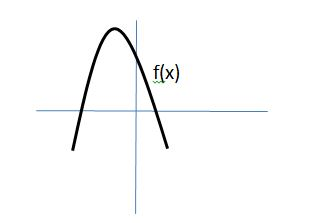The graph of f(x) is shown above. If f(x) = ax2 + bx + c, where a, b, and c are real numbers, then which of the following must be true:

I. a < 0

II. c < 0

III. b2 – 4ac < 0

I only

II only

I and II only

II and III only

I and III only

I only

Explanation:

Let's examine I, II, and III separately.

Because the parabola points downward, the value of a must be less than zero. Thus, a < 0 must be true.

Next, let's examine whether or not c < 0. The value of c is related to the y-intercept of f(x). If we let x = 0, then f(x) = f(0) = a(0) + b(0) + c = c. Thus, c is the value of the y-intercept of f(x). As we can see from the graph of f(x), the y-intercept is greater than 0. Therefore, c > 0. It is not possible for c < 0. This means choice II is incorrect.

Lastly, we need to examine b2 – 4ac, which is known as the discriminant of a quadratic equation. According to the quadratic formula, the roots of a quadratic equation are equal to the following:Notice, that in order for the values of x to be real, the value of b2 – 4ac, which is under the square-root sign, must be greater than or equal to zero. If b2 – 4ac is negative, then we are forced to take the square root of a negative number, which produces an imaginary (nonreal) result. Thus, it cannot be true that b2 – 4ac < 0, and choice III cannot be correct.

Only choice I is correct.

### Example Question #675 : Sat Mathematics

Which of the following functions represents a parabola that has a vertex located at (–3,4), and that passes through the point (–1, –4)?

f(x) = 2x2 – 12x – 14

f(x) = x2 + 6x + 13

f(x) = x2 – 5

f(x) = 2x2 + 4x – 2

f(x) = –2x2 – 12x – 14

f(x) = –2x2 – 12x – 14

Explanation:

Because we are given the vertex of the parabola, the easiest way to solve this problem will involve the use of the formula of a parabola in vertex form. The vertex form of a parabola is given by the following equation:

f(x) = a(x  h)2 + k, where (h, k) is the location of the vertex, and a is a constant.

Since the parabola has its vertex as (–3, 4), its equation in vertex form must be as follows:

f(x) = a(x – (–3)2 + 4 = a(x + 3)2 + 4

In order to complete the equation for the parabola, we must find the value of a. We can use the point (–1, –4), through which the parabola passes, in order to determine the value of a. We can substitute –1 in for x and –4 in for f(x).

f(x) = a(x + 3)2 + 4

–4 = a(–1 + 3)2 + 4

–4 = a(2)2 + 4

–4 = 4a + 4

Subtract 4 from both sides.

–8 = 4a

Divide both sides by 4.

a = –2

This means that the final vertex form of the parabola is equal to f(x) = –2(x + 3)2 + 4. However, since the answer choices are given in standard form, not vertex form, we must expand our equation for f(x) and write it in standard form.

f(x) = –2(+ 3)2 + 4

= –2(x + 3)(x + 3) + 4

We can use the FOIL method to evaluate (+ 3)(+ 3).

= –2(x2 + 3x + 3x + 9) + 4

= –2(x2 + 6x + 9) + 4

= –2x2 – 12x – 18 + 4

= –2x2 – 12x – 14

The answer is f(x) = –2x2 – 12x – 14.

### Example Question #261 : New Sat

The parabolas of the functionsand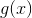on the coordinate plane have the same vertex.

If we define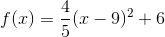, which of the following is a possible definition of?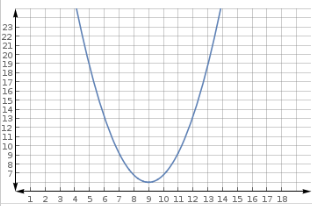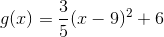None of the other responses gives a correct answer.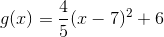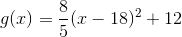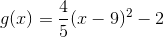Explanation:

The definition ofis given in the vertex form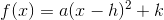,

so the vertex of its parabola is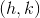. The graphs ofandare parabolas with the same vertex, so they must have the same values forandFor the function,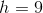and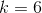.Of the five choices, the only definition ofthat has these same values, and that therefore has a parabola with the same vertex, is.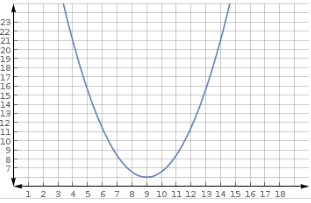To verify, graph both functions on the same grid.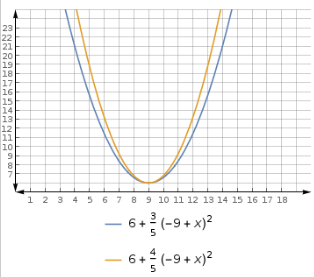### All SAT Math Resources